# The Excel TEXT FunctionClaudia Buckley

Take your Excel skills to the next level with our comprehensive (and free) ebook!

The Excel TEXT function allows you to convert numbers to Excel text format using Excel’s format codes. You’ll find it useful whenever you want to display numbers in a more readable format. Sometimes you might even want to combine numbers with text or symbols.

For instance, you may have today’s date entered in a cell (see A1 below), and you need this date to become part of a text string in cell A7.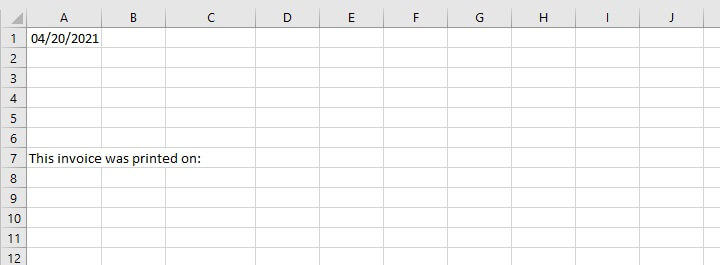If you join the date element to a text string (in this case, we’ve used the & symbol), Excel becomes confused and drops the date formatting that was applied to cell A1 because the entire cell is automatically given a General number format.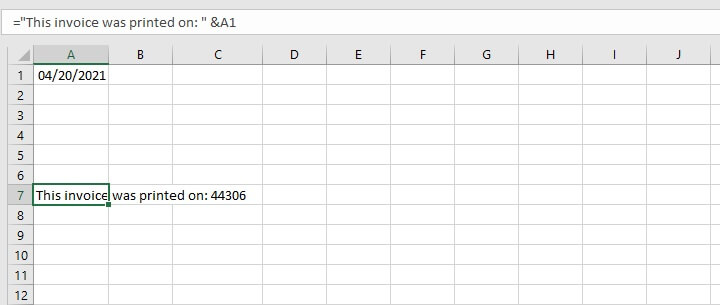The Excel TEXT function can be used to convert numbers to text in these and other situations.

## Syntax

The syntax of the TEXT function is:

````=TEXT(value, format_text)`
```
• Value - is the numeric value that you want to be converted into text.
• Format_text - is the format that you would like to apply to the value.

Note that the format_text argument must be written in double quotation marks.

## Return value

The return value is a text string in the stated format.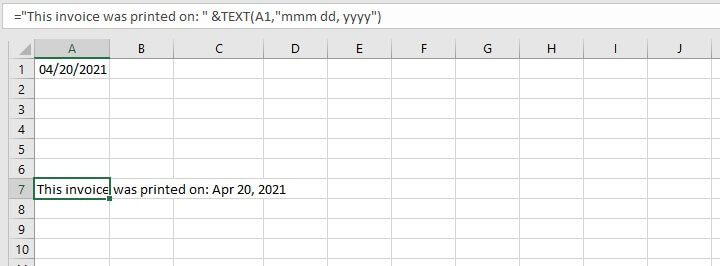Though you can use explicit numerical values inside the formula, it’s usually a better idea to make reference to a cell that carries that value.

This is because if you need to make reference to the value later for another calculation, Excel may give an unexpected result because it doesn’t interpret the value well, or because of how it was asked to handle it. Notice the two different results in the examples below.

The following formula was typed into cell B1:

``=TEXT(0.598,"#%")``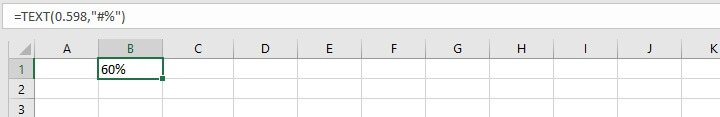The output is 60%.

And now let’s put the value 0.598 in cell A2 and refer to it using the same format_text argument.

````=TEXT(A2,"#%")`
```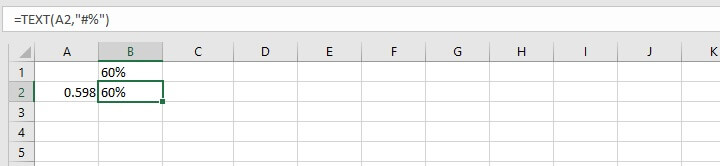The result is exactly the same as before. But if we perform a calculation on the values in column B, do they carry the same value? Let’s add 1% to each of these values:

B1+1% and

A2+1%.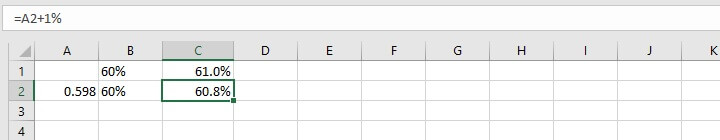If the original values are important, the best practice is to keep them in their original form, but reference them when using the TEXT function to do the conversion.

Naturally, to use the TEXT function requires familiarity with the various format codes.

## Commonly-used format codes

Below is a non-exhaustive list of codes that may be used in the format_text argument of the TEXT function.

The first table shows symbols that are used to control the appearance of general numbers.

Symbol

Description

Example

0 (zero)

Forced digit. Zeros are displayed if there is no digit in that position.

=TEXT(123,“0000.00”)

Result: 0123.00

# (hash)

Digit placeholder. Extra zeros are not displayed.

=TEXT(12.34, “#.#”)

Result: 12.3

?

Digit placeholder. Useful for alignment of decimal points in a column.

=TEXT(12.34, “???.?”)

=TEXT(456.7, “???.?”)

Result:   12.3

Result: 456.7

This next table shows symbols that are used for date and time formatting.

Symbol

Description

Examples

d

Day of month, or day of week

d - one or two-digit number representing day of month (1-31)

=TEXT(2, “d”)

Result: 2 [meaning the 2nd day of the month]

dd - two-digit number with a leading zero representing day of month (01 to 31)

=TEXT(2, “dd”)

Result: 02 [meaning the 2nd day of the month]

ddd - three-letter abbreviation (Mon to Sun)

=TEXT(2, “ddd”)

Result: Mon

dddd - full name of day of week (Monday to Sunday)

=TEXT(2, “dddd”)

Result: Monday

m

Month

m - one or two-digit number representing month (1 to 12)

=TEXT(6, “m”)

Result: 6 [meaning the 6th month of the year]

mm - two-digit number with a leading zero representing month (01 to 12)

=TEXT(6, “dd”)

Result: 06 [meaning the 6th month of the year]

mmm - 3-letter month abbreviation (Jan to Dec)

=TEXT(6, “ddd”)

Result: Jun

mmmm - full name of month (January to December)

=TEXT(6, “dddd”)

Result: June

y

Year

yy - two-digit year number

=TEXT(02/07/2009, “mmm yy”)

Result: Feb 09

yyyy - four digit number (e.g. 2006, 2016)

=TEXT(02/07/2009, “mmmm yyyy”)

Result: February 2009

h

Hour

h - one or two-digit number representing hour (1 to 24)

=TEXT(6:37 PM, “h”)

Result: 18

hh - two-digit number representing hour (01 to 24)

=TEXT(6:37 AM, “hh”)

Result: 06

m

Minute

m - one or two-digit number representing minute (1 to 60)

=TEXT(

mm - two-digit number representing minute (01 to 60)

s

Second

s - one or two-digit number without a leading zero (1 to 60)

ss - two-digit number with a leading zero (01 to 60)

AM/PM

Time represented as a 12-hour clock, followed by "AM" from midnight until before noon, or "PM" from noon until before midnight.

a/p

Time represented as a 12-hour clock, followed by "a" from midnight until before noon, or "p" from noon until before midnight.

It’s important to note the following:

• The above characters are not case-sensitive. For example, the code M is interpreted in the same way as m.
• The m or mm code should appear immediately after the h or hh code (or it should be immediately before the ss code). Otherwise, Excel will display the month instead of minutes.

The following characters may also be used in the format code. They will be displayed as entered once they are entered within double quotation marks.

Character

Name

+

Plus sign

-

Minus sign

( )

Parenthesis

:

Colon

{ }

Curly brackets

< >

Less-than and greater than signs

=

Equal sign

/

Forward slash

!

Exclamation point

&

Ampersand

~

Tilde

Space character

Period (decimal point)

Comma (thousands separator)

Below are some useful examples and applications of the TEXT function.

If you attempt to type the number 0123456789 in an Excel cell, the leading zero will be dropped because Excel automatically formats this as a number, and it knows that leading zeros have no value.

But what if that zero is important? Maybe this is an account number and you need all ten places to be shown, including the leading zero. Using the TEXT function with format codes is one solution to this problem.

``=TEXT(A2, “0000000000”)``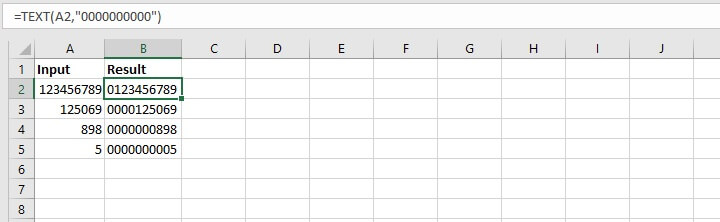As you can see, using the zero placeholder ensures that the output will have the desired number of digits displayed in the result cell.

## Formatting telephone numbers

Parentheses and dashes may be used to format telephone numbers the way you want. To display a phone number in the following format

(area code) prefix - line

the format would simply be

````TEXT(value, “(###) ### - ####”)`
```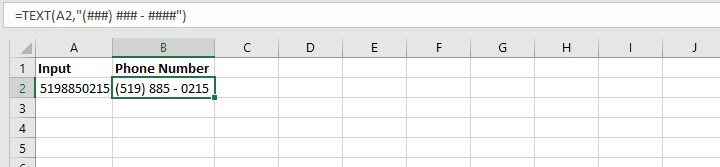## Formatting time

We can use the AM/PM format to combine time and text into a more readable format.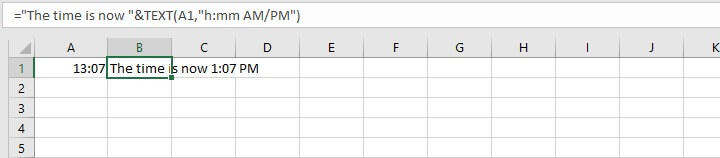Of course, we can concatenate as many times as necessary to accomplish our objective, as shown below.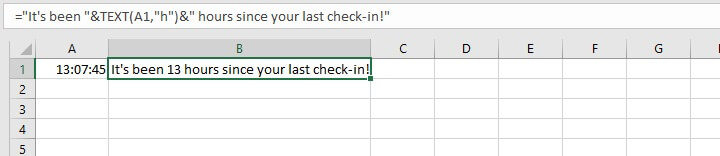## Troubleshooting the TEXT function

If you encounter any errors when working with the Excel TEXT function, here are the three main things to check for:

1. Ensure that the format_text argument is entered within double quotes. Otherwise, you will get a #NAME? error.
2. Numeric placeholder symbols (0, #, ?) cannot be combined with time placeholders or text within the same enclosure. For example, =TEXT(A1, “#h”) will throw a #VALUE! error, but =TEXT(A1,"#")& " hours" is valid.
3. Pay attention to local computer settings if you get date and/or time errors, bearing in mind that the d, m, y codes are English-language specific.

## Conclusion

The TEXT function isn’t the only way to get numbers to display the way we want. We can usually find the most common formats in Excel’s built-in formats in the Format Cells options. However, it’s nice to know that we do have the option to customize when the solution doesn’t come ready-made.

Learn how to use Excel to solve your needs today by trying one of our courses. You can try the free Excel in an Hour course for some quick basics.

But if you really want to make the best of Excel we recommend the comprehensive Basic and Advanced course. It will take you from all the basics to those advanced topics that you need to know to really take advantage of the power of Excel.